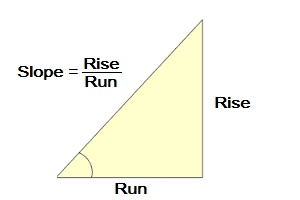## Slope Percentage Calculator

Find the percent of a slope (rise over run) with this Slope Percentage Calculator.

Rise:
Run:
Fill in amount of rise and the amount of run on a slope to find
the percent of slope.

## How do you find the percent of slope using rise over run?

To find the percentage of a slope, use this slope percent formula:
Slope Percent = (Amount of Rise / Amount of Run) x 100

Example: The angle percent of a slope with rise of 6 units and run
of 10 units is:

Angle Percentage = (6 /10) x 100

Calculated out this gives an angle percentage of 60%.